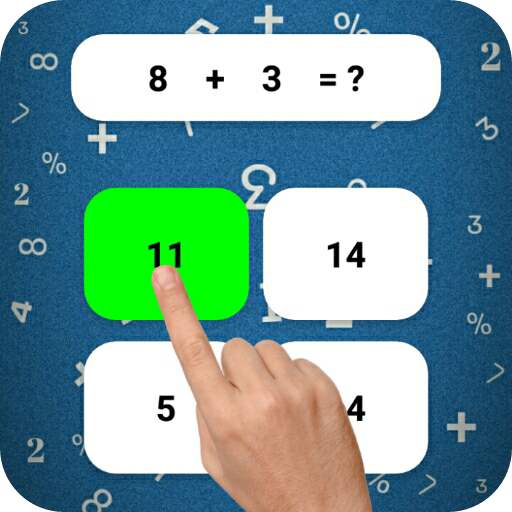# Math Games, Learn Plus, Minus, Multiply & Division on PC/Mac

Author: Jigar Education Hub

Score: 4.2

OS: Windows, OS X, 4.4 +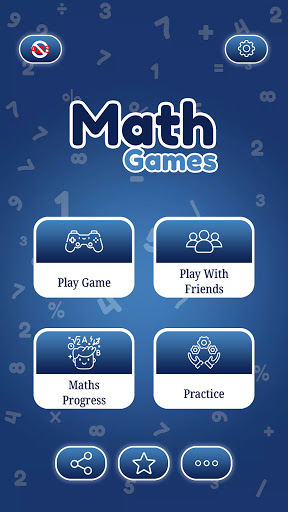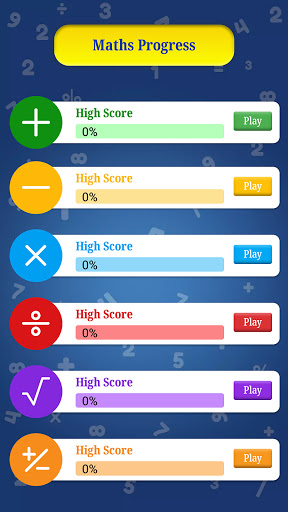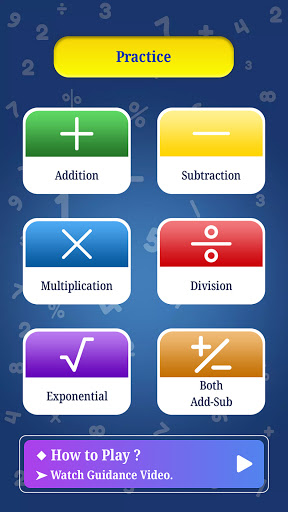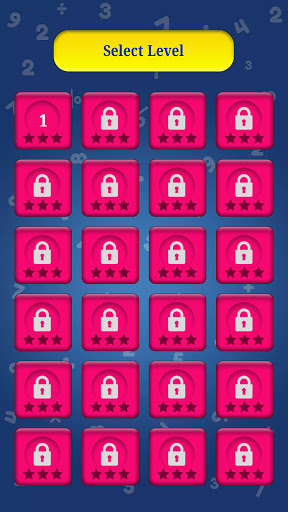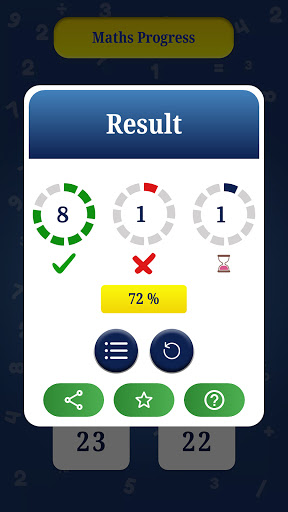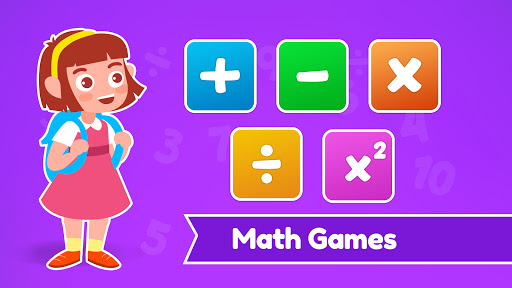##### About Math Games, Learn Plus, Minus, Multiply & Division
LEADING Math Game on Play Store
HIGHLY RATED and UPDATED Game

Are you looking for the BEST math game on the play store?
Do You want to DEVELOP your BRAIN & MATHEMATICS CALCULATION POWER?

Math Games, Learn Plus, Minus, Multiply & Division by Jigar Education Hub is the right math app for you.

The goal behind making this game for the Jigar Education Hub team is that you can easily learn mathematics by playing the game. With this educational app parents, teachers and educators can help students to learn faster.

Math Games
Learn math's all operation with fun
➖ Subtraction
✖️ Multiplication
➗ Division
√ Square (root )
➕,➖ Mixed operations
👬 Play with friend

Benefits of Using Math Games
• Meets Mathematics Standards
• Easily Linked to Any Mathematics Textbook
• Offers Multiple Assessment Opportunities
• Meets the Needs of Diverse Learners (UA)
• Supports Concept Development in Math
• Encourages Mathematical Reasoning
• Engaging (maintains interest)
• Repeatable (reuse often & sustain involvement
• Open-Ended (allows for multiple approaches & solutions)
• Easy to Prepare
• Easy to Vary for Extended Use & Differentiated Instruction
• Improves Basic Skills
• Enhances Number and Operation Sense
• Encourages Strategic Thinking
• Promotes Mathematical Communication
• Promotes Positive Attitudes Toward Math
• Encourages Parent Involvement

Features
• Addition Games: Simple Addition game to learn math in a fun way.
• Subtraction games: Subtracting numbers to solve the equations.
• Multiplication Games: Multiplication Table is a useful tool for everyone every day.
• Division Games: Enjoy surpassing the division game and learn the division's table.
Math games for All: Improve your math skills for various mathematical operations like addition, subtraction, multiplication, and division.
Brain Training: Here are 60 levels, All levels are suitable for adults and all.
• challenge game: challenge your friend 2 player game.
Cool math games: Cool Math Games provides all kinds of interesting and fun games for players of all ages and skill levels.
• puzzles in maths: Try using math puzzles to get students strategically thinking about math.
• Educational games: Educational games are games that are designed to help people learn about certain subjects.
• Math learning: its learning games help you to learn cool math examples.
Multiplication Table: You can learn all the Multiplication tables from 1 to 20 by playing this game.
• Math Practice: By doing many basic examples Practices, you will have much impression of all types of examples.
Math Exercises : Learn Add, Subtract, Multiply & Divide

What is a math game ?
• The most effective math games are those in which the structure and rules of the games are based on mathematical ideas, and winning a game is directly related to understanding mathematics.

• This game is best for him who is looking for "cool math games"

Support 15+ Multiple Languages
• English, Japanese, Chinese Simplified, Chinese Traditional, Hindi, Russian, Korean, German, Spanish, Portuguese, Indonesian, French, Italian, Malay, Turkish, Thai.